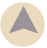# Glaze Weight to Percentage Calculator

If a glaze recipe is formulated for a specific batch size, this calculator can convert it to a precentage formula: each raw material in the glaze is converted from its weight to its corresponding percentage.

Once converted to percentages, the Glaze Batch Size Calculator can be used to formulate other sized batches.

(optional)

## Raw Materials in Glaze

Indredient Amount
1.
2.
3.
4.
5.
6.
7.
8.
9.
10.

### More Glaze Calculators